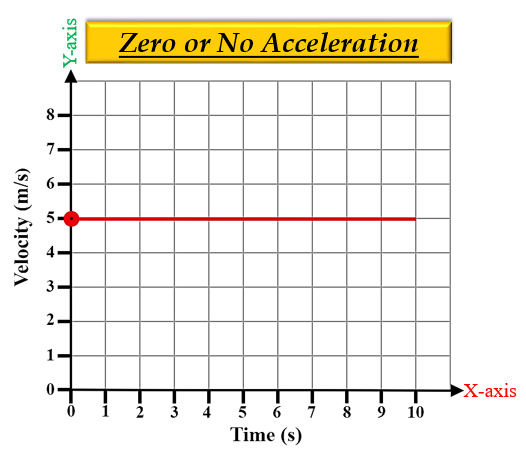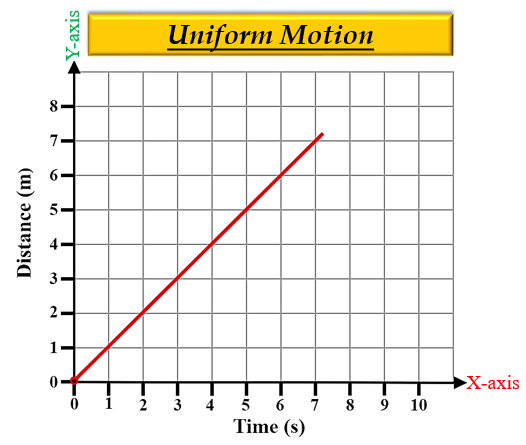# Velocity-time graph for the motion of an object in a straight path is a straight line parallel to the time axis. (a) Identify the nature of motion of the body. (b) Find the acceleration of the body. (c) Draw the shape of distance-time graph for this type of motion.

Velocity-time graph for the motion of an object in a straight path is a straight line parallel to the time axis.(a) The nature of motion of the body will be constant or uniform as the acceleration is zero.

When the velocity of the body remains constant, then it means the speed and the direction of the body is not changing with respect to time. Hence, there is zero or no acceleration.

So, the velocity-time graph of an object in a straight path is a straight line parallel to the x-axis (time axis).

(b) We know that, acceleration is the rate of change of velocity. Here, from the graph we can see that velocity is not changing, remains constant. Hence, the acceleration will be zero.

(c) The shape of distance-time graph for this type of motion (uniform motion) is given below:

Uniform motion- A body is said to be in uniform motion if it travels equal distances in equal intervals of time, no matter how small these time intervals may be. In other words, the distance travelled is directly proportional to time. Thus, a distance-time graph for uniform motion will be a straight line.Updated on: 10-Oct-2022

5K+ Views